## MTH213 Foundations of Elementary Math 3

### Fun with Statistics.Notes on Statistics
Spring 2020

#### Chapter 7 Statistics

##### Descriptive Statistics part 1
• Types of Graphs
• Line Plots aka Dot Plots
• Stem and Leaf Plots
• Pictographs
• Bar or Column Charts
• Pie Charts
• Histograms and Frequency Table
• Line Graphs, time series
• Scatter Plots
• Trend line
• Curves of Best Fit
• Regression Equations
• Correlation
• Linear
• Exponential
• Power
##### Math Activity: Build Basic Charts

Purpose: Explore building charts appropriate to the data.

Materials: Imagination, paper, color.

Directions: Find data for each of the chart types below. For each chart you will need a table to summarize the data, then build the chart. When you are done, take a picture of each page to upload.

Keep in mind that some charts are not appropriate for some data. Proceed with care.

Look through section 7.1 for ideas. Look online for ideas. Look around your house, inside and out. Think about your family and friends. What can you categorize into two or more groups and count? If you do find a good idea online, then personalize it or just reproduce it.

1. Pie Chart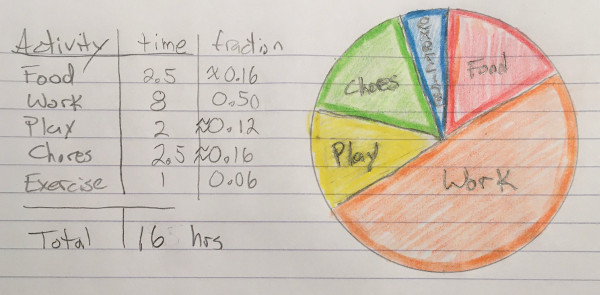2. Bar Chart3. Pictograph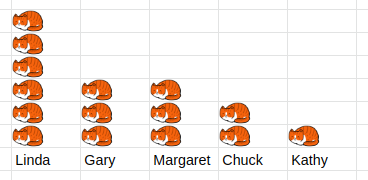4. Line Plot aka Dot Plot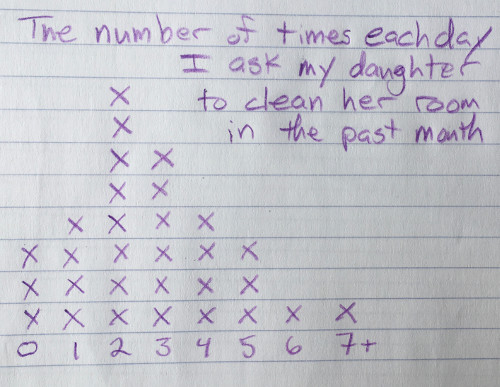5. Line Graph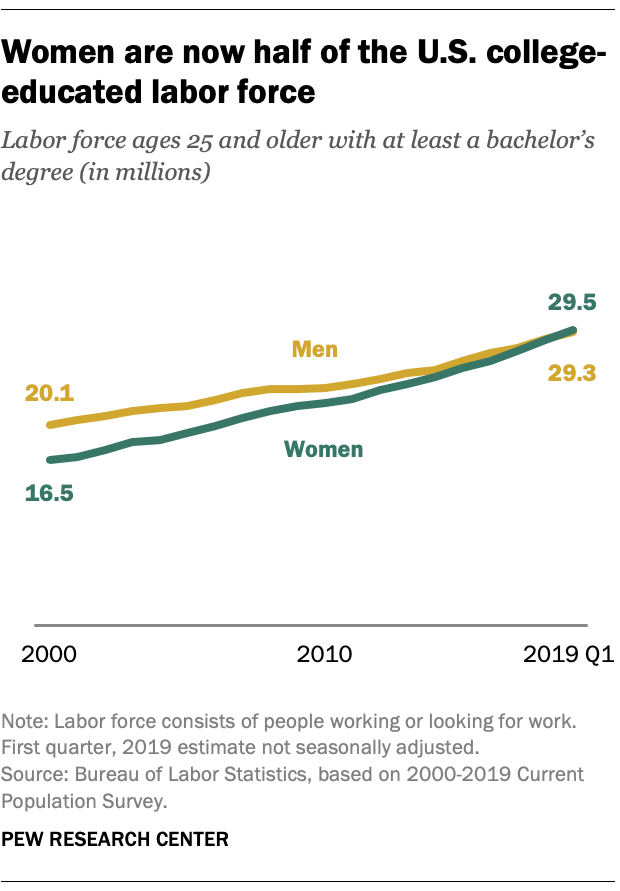I wouldn't turn this graph in for my assignment. I would need to reproduce it and cite my source.
##### Math Activity: Build a Histogram

Purpose: Explore building a histogram based on a large data set.

Materials: Imagination, paper, color.

Directions: Find or make a data set with at least 30 entries. StatKey has some good data set to think about.

1. Raw data
2. Grouping decision 1
3. Frequency table 1
4. Histogram 1
5. Grouping decision 2
6. Frequency table 2
7. Histogram 2
##### Math Activity: Build a Scatter Plot and Trend Line

Purpose: Explore bivariate data and correlation.

Materials: Imagination, paper, color.

Directions: Find or make a bivariate data set with at least 10 entries.
Spurious Correlations has some interesting? examples, as does StatKey.

1. Definitions of each variable
2. Table of data
3. Scatter plot, well labeled
4. Line drawn through points that "fits" trend
5. Equation of line
6. Is the line a good fit? Why?
7. Use the equation to make a prediction? Explain the prediction.

#### Chapter 7 Statistics

##### Descriptive Statistics part 2
• Descriptive Statistics the science of describing data with charts and summary calculations.
• Measures of Center
• Mean
average = "sum"/"count"
• Median
middle value when ranked, position = (n+1)/2
the value where 50% of the data is less than or equal.
• Mode
most frequently occuring
• Range = max - min
• Quartiles
The first quartile, Q_1 is the value where 25% of the data is less than or equal.
The third quartile, Q_3 is the value where 75% of the data is less than or equal.
• InterQuartile Range = Q_3-Q_1
• Standard Deviation
a measure of average spread from the mean.
• Box and Whisker Plots
a graph of the 5-number summary, min, Q_1, median, Q_3, max
• Data Distribution
a graph of description of the center and spread of the data
Using spreadsheets to do descriptive statistics.

#### Chapter 7 Statistics

##### Inferential Statistics
• Sample vs Population
• Statistic vs Parameter
• Random Sampling
each group of size n in a population has the same chance of being selected as any other group of size n.
• Stratified Sampling
• Data Distributions
• Skewed Distributions

The skew is to the tail.

• Symmetrical
mean ~~ median• Skewed left
mean < median• Skewed right
mean > median• BiModal• Bell Shaped• Normal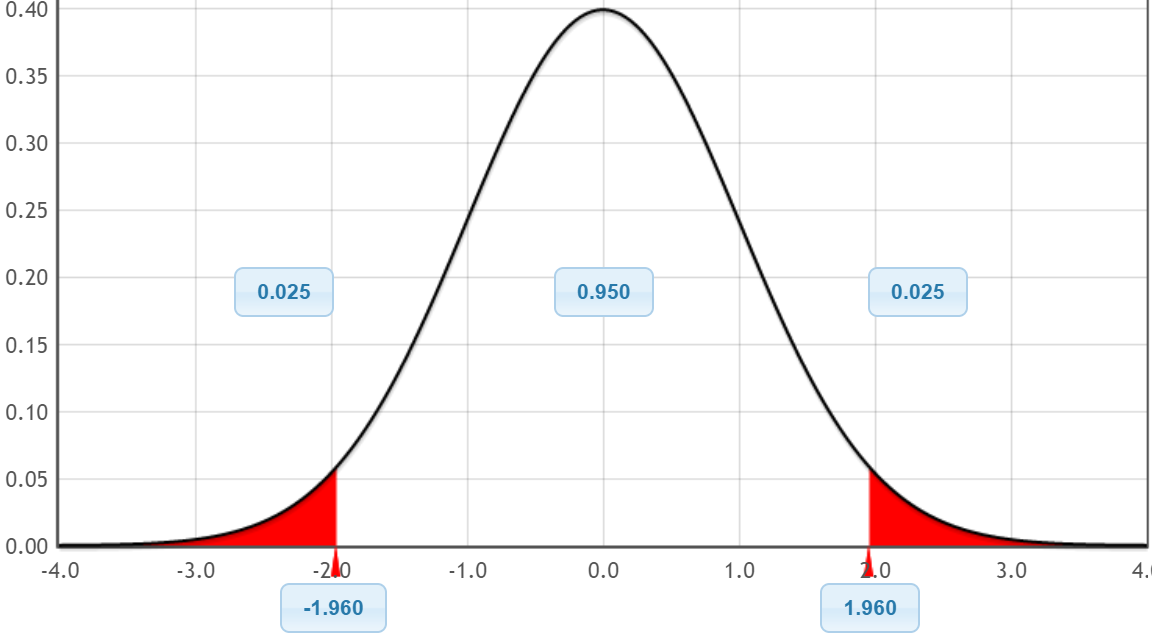• Measures of Relative Standing
• Percentiles
• z-scores
• Rare events
• Simulations